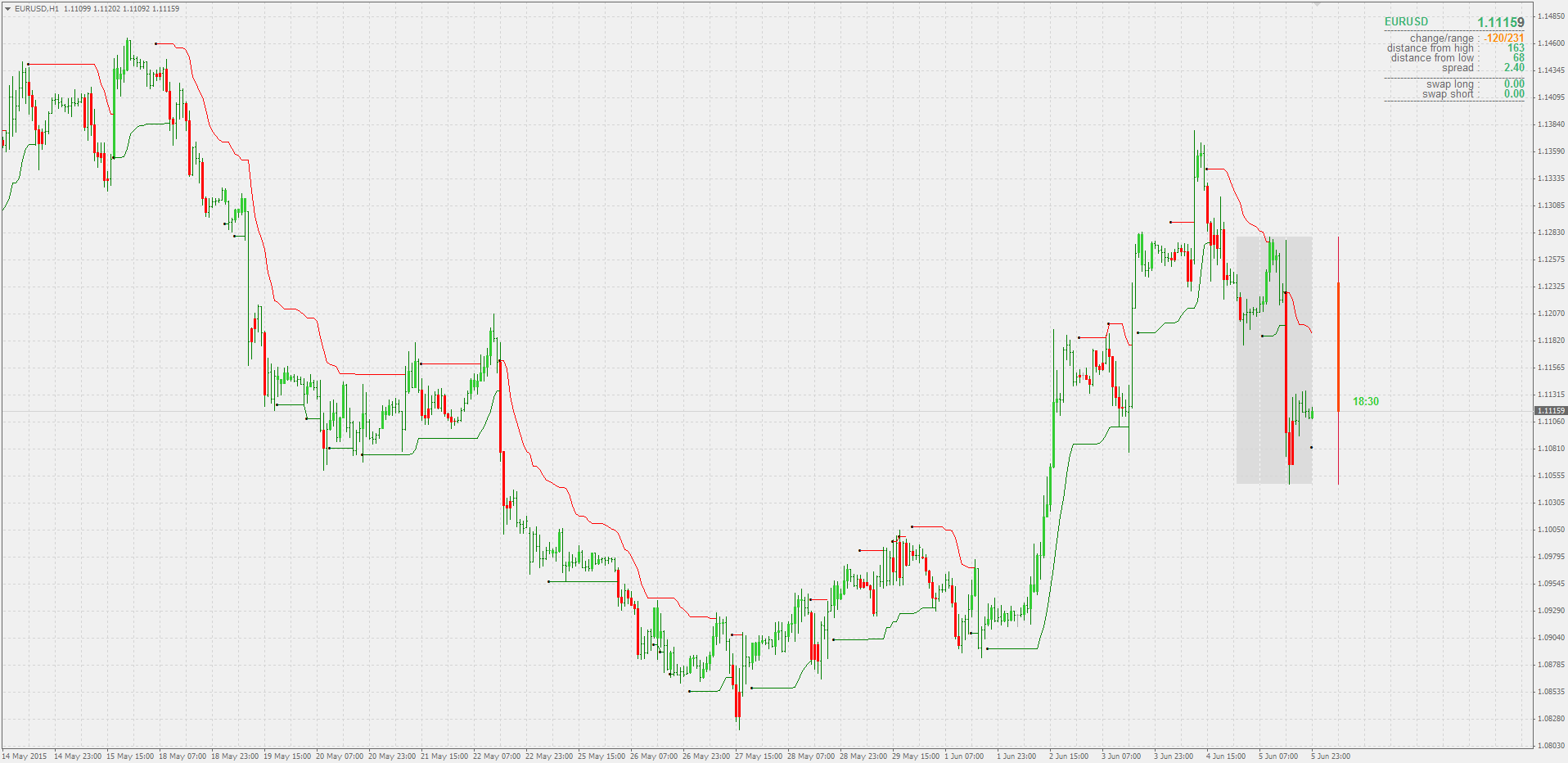# Indicators with alerts/signal - page 1126154658

samuelkanu:

Some signals can overlap (even appear at the same time - see the lines) :Files:3231

Some signals can overlap (even appear at the same time - see the lines) :I would like to know how to trade those lines. The overlapping seems to be causing false signals4412

I would like to know how to trade those lines. The overlapping seems to be causing false signals

with overlapping orders obviously...35

I cud not find option to upload, so here is the indicator :35

//+------------------------------------------------------------------+

//| smoothed rsi |

//+------------------------------------------------------------------+

#property indicator_separate_window

#property indicator_buffers 5

#property indicator_color1 Gray

#property indicator_color2 LimeGreen

#property indicator_color3 LimeGreen

#property indicator_color4 Orange

#property indicator_color5 Orange

#property indicator_width2 3

#property indicator_width3 3

#property indicator_width4 3

#property indicator_width5 3

#property indicator_minimum 0

#property indicator_maximum 100

//

//

//

//

//

extern int Length = 14;

extern int Price = PRICE_CLOSE;

extern double LevelUp = 70;

extern double LevelDown = 30;

extern double SmoothLength = 20;

//

//

//

//

//

double rsi[];

double rsiUa[];

double rsiUb[];

double rsiDa[];

double rsiDb[];

double prc[];

double trend[];

//+------------------------------------------------------------------+

//| |

//+------------------------------------------------------------------+

//

//

//

//

//

int init()

{

IndicatorBuffers(7);

SetIndexBuffer(0,rsi);

SetIndexBuffer(1,rsiUa);

SetIndexBuffer(2,rsiUb);

SetIndexBuffer(3,rsiDa);

SetIndexBuffer(4,rsiDb);

SetIndexBuffer(5,prc);

SetIndexBuffer(6,trend);

Length = MathMax(Length ,1);

string PriceType;

switch(Price)

{

case PRICE_CLOSE: PriceType = "Close"; break; // 0

case PRICE_OPEN: PriceType = "Open"; break; // 1

case PRICE_HIGH: PriceType = "High"; break; // 2

case PRICE_LOW: PriceType = "Low"; break; // 3

case PRICE_MEDIAN: PriceType = "Median"; break; // 4

case PRICE_TYPICAL: PriceType = "Typical"; break; // 5

case PRICE_WEIGHTED: PriceType = "Weighted"; break; // 6

}

//

//

//

//

//

SetLevelValue(0,LevelUp);

SetLevelValue(1,LevelDown);

if (SmoothLength>1) addName = "smoothed ";

return(0);

}

int deinit()

{

return(0);

}

//+------------------------------------------------------------------+

//| |

//+------------------------------------------------------------------+

//

//

//

//

//

int start()

{

int counted_bars=IndicatorCounted();

if(counted_bars<0) return(-1);

if(counted_bars>0) counted_bars--;

int limit = MathMin(Bars-counted_bars,Bars-1);

//

//

//

//

//

//

if (trend[limit]== 1) CleanPoint(limit,rsiUa,rsiUb);

if (trend[limit]==-1) CleanPoint(limit,rsiDa,rsiDb);

for(int i=limit; i >= 0; i--)

{

double price = iMA(NULL,0,1,0,MODE_SMA,Price,i);

if (SmoothLength>1)

price = iSsm(price,SmoothLength,i);

prc = price;

}

for(i=limit; i >= 0; i--)

{

rsi = iRSIOnArray(prc,0,Length,i);

rsiUa = EMPTY_VALUE;

rsiUb = EMPTY_VALUE;

rsiDa = EMPTY_VALUE;

rsiDb = EMPTY_VALUE;

//

//

//

//

//

trend = trend;

if (rsi>LevelUp) trend= 1;

if (rsi<LevelDown) trend=-1;

if (rsiLevelDown) trend= 0;

if (trend == 1) PlotPoint(i,rsiUa,rsiUb,rsi);

if (trend == -1) PlotPoint(i,rsiDa,rsiDb,rsi);

}

return(0);

}

//------------------------------------------------------------------

//

//------------------------------------------------------------------

//

//

//

//

//

void CleanPoint(int i,double& first[],double& second[])

{

if ((second != EMPTY_VALUE) && (second != EMPTY_VALUE))

second = EMPTY_VALUE;

else

if ((first != EMPTY_VALUE) && (first != EMPTY_VALUE) && (first == EMPTY_VALUE))

first = EMPTY_VALUE;

}

//

//

//

//

//

void PlotPoint(int i,double& first[],double& second[],double& from[])

{

if (first == EMPTY_VALUE)

{

if (first == EMPTY_VALUE) {

first = from;

first = from;

second = EMPTY_VALUE;

}

else {

second = from;

second = from;

first = EMPTY_VALUE;

}

}

else

{

first = from;

second = EMPTY_VALUE;

}

}

//------------------------------------------------------------------

//

//------------------------------------------------------------------

//

//

//

//

//

double workSsm[];

#define _price 0

#define _ssm 1

double workSsmCoeffs[];

#define _period 0

#define _c1 1

#define _c2 2

#define _c3 3

#define Pi 3.14159265358979323846264338327950288

//

//

//

//

//

double iSsm(double price, double period, int i, int instanceNo=0)

{

if (ArrayRange(workSsm,0) !=Bars) ArrayResize(workSsm,Bars);

if (ArrayRange(workSsmCoeffs,0) < (instanceNo+1)) ArrayResize(workSsmCoeffs,instanceNo+1);

if (workSsmCoeffs[_period] != period)

{

workSsmCoeffs[_period] = period;

double a1 = MathExp(-1.414*Pi/period);

double b1 = 2.0*a1*MathCos(1.414*Pi/period);

workSsmCoeffs[_c2] = b1;

workSsmCoeffs[_c3] = -a1*a1;

workSsmCoeffs[_c1] = 1.0 - workSsmCoeffs[_c2] - workSsmCoeffs[_c3];

}

//

//

//

//

//

i = Bars-i-1;

int s = instanceNo*2;

workSsm[s+_price] = price;

workSsm[s+_ssm] = workSsmCoeffs[_c1]*(workSsm[s+_price]+workSsm[s+_price])/2.0 +

workSsmCoeffs[_c2]*workSsm[s+_ssm] +

workSsmCoeffs[_c3]*workSsm[s+_ssm];

return(workSsm[s+_ssm]);

}154658

Abhinav:

Abhinav

Version with alerts, multi time frames and some extras posted here : https://www.mql5.com/en/forum/general65

Can you work on the indicator of for me if possible?

bars_ob_ident.mq4

Files:135

Are you guys familiar with Nadex? If you guys are, i was wondering if you guys could create an indi for me. Nadex have a binary 5 min expiry with 5 strike price.

Every last 10 second of the current 5 min candle, where ever price is at that moment a new set of 5 strike price will be calculated from it.

The middle strike price will always be at the last 10 sec price. The two strike price above and below the middle strike price are 3 pips apart.

I was wondering if you guys could create a 5 horizontal lines that will draw out these strike price.

If doing at the last 10 sec is not possible could you guys do it on the closing of the candle. could guys add the option of changing the pips and also change the expiry time if possible. Nadex has other expiry that i would like to test out like 20 min expiry and up.

Really much appreciated..And thank very much in advance..111